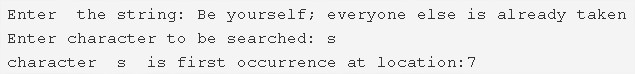# C Program To Find First Occurrence Of A Character In A String

C program to find the first occurrence of a character in a given string – In this article, we will brief in on the multiple methods to find the first occurrence of a character in a given string in C programming.

Suitable examples and sample programs have also been added so that you can understand the whole thing very clearly. The compiler has also been added with which you can execute it yourself.

The methods used in this piece are as follows:

• Using Standard Model
• Using Function
• Using Recursion

A string is nothing but an array of characters. The value of a string is determined by the terminating character. Its value is considered to be 0.As you can see with this example, you need to enter a string first up.

The string entered here is “Be yourself; everyone else is already taken”.

After that, you need to specify which character you need to search in the given string.

The character that is searched here is ‘s’.

Hence, after checking, it is confirmed that ‘s’ comes at the 7th spot in the string.

Thus, doing the same in C programming is as follows:

## Using Standard Method

1. Read the entered string using gets(s) and read the character to be searched using getchar() function and store the char into the variable c.

2) For loop iterates through string with the structure for(i=0;s[i];i++)

Compare the character c with s[i]

If character c matches with s[i] then initialize k=1 and come out of the loop.

3) If k=1 then print the character first occurrence at the location i.

If k!=1 then print the character is not in the string.

Output:

### Using Function

1. The main() function calls the check() function by passing string and char as arguments to the function.

2) The function compares the char c with the elements of the string using for loop for(i=0;s[i];i++).

a) If c match with s[i] then initialize k=1 and break the loop.

b) If k=1 then the function returns i value otherwise return -1. The variable i indicates the first occurrence location of the character in the string.

3) The returned value initialized to n. The main() prints the first occurrence of the character in the given string.

If n>-1 then the main() function prints the first occurrence of the character in the given string otherwise it prints the character is not in the string.

Output:

#### Using Recursion

1. The function check(char *s,char c)

a) Returns -1 if there is no character has existed at s[i].

b) If s[i] existed then

Compare s[i] with the character c. If both are equal then return i value.

else increase the i value and then call the function itself.

The function calls itself recursively until the character to be searched is equal to one of the characters of the string.

2) If the returned value is >-1 then print the location of the character where it occurs first in the string. Otherwise, print the character is not available in the string.

Output: